# What is a scalar field 1

1. Scalar and vector fields
2. Nabla operator
4. divergence
5. rotation

### Scalar and vector fields

A field is generally understood to mean a space in which any physical quantity is assigned to each point P (x; y; z).
If it is an undirected quantity (scalar), then one speaks of a scalar field. E.g .: temperature, density, pressure. This paper uses the notation f (x, y, z). (In theory, of course, a different number of variables is also possible.)
If it is a directed quantity (vector), then one speaks of a vector field. E.g .: flow, gravity, magnetic lines, electric field. This paper uses the notation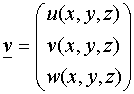used. (A different number of components or variables is possible.)

### Nabla operator

The Nabla operator is a formal vector whose components consist of the partial differential operators.

Definition: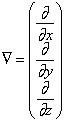(Analogous for other dimensions)

Let f (x, y, z) be a scalar field, the gradient of f is a vector which points in the direction of the greatest change in f at point P (x; y; z) and whose magnitude is equal to this greatest change.

Definition: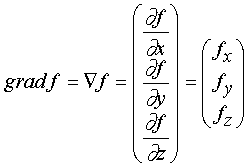The gradient is thus normal to the level surface through P. If a potential field has been given, the gradient always points in the direction in which a particle would move if it were brought into the field at P.

### divergence

The divergence is for a vector fieldv calculated and is itself a scalar. For example, it indicates for a flow field whether more liquid flows out of an infinitesimal volume, P (x; y; z) than flows into it.

Definition: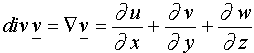If the divergence is positive, there is a source in P; if it is negative, there is a sink in P. If the divergence is 0, then just as much liquid flows into the volume as it flows out.

### rotation

The rotation is for a vector fieldv computes, and is itself a vector.

Definition: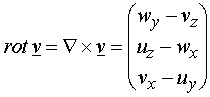If you consider an infinitesimal volume in the vector field, the rotation vector indicates how strongly and about which axis of rotation the volume rotates. If the rotation is 0, then the vector field is free of eddies.

back to partial derivative and total differential
further to the Jacobi matrix
Mathematical basics overview